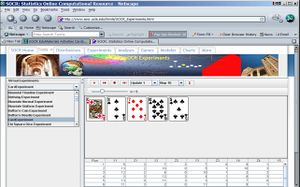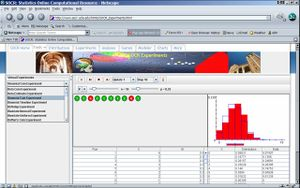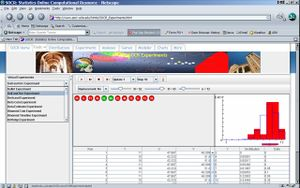# SOCR EduMaterials Activities DieCoin

(diff) ← Older revision | Latest revision (diff) | Newer revision → (diff)

## This is a heterogeneous Activity that demonstrates different settings for sampling drawing synergetic parallels between empirical and theoretical probability calculations

• Exercise 1: Supose a random card is drawn from a standard well-shuffled deck of 52 playing cards. What is the theoretical probability of the event A = {the drawn card is a king or a club}? In this applet an outcome of a King is recorded in the first variable Y and corresponds to Y=13; the second variable Z corresponds to the suit of the card with a Club, ♣, represented by Z=0. Using the SOCR Card-Experiment perform 20 experiments and determine the proportion of the outcomes the event A was observed. This would correspond to the emperical probability (the chance) of the event A. How close are the observed and the theoretical proabilities for the event A? Would the discrepancy between these increase or dicrease if the number of hands drawn increases? First do the xperiment and then report you findings!• Exercise 2: Suppose we need to validate that a coin given to us is fair. We toss the coin 6 times independently and observe only one Head. If the coin was fair P(Head)=P(Tail)=0.5 and we would expect about 3 Heads and 3 Tails, right? Under these fair coin assumptions what is the (theoretical) probability that only 1 Head is observed in 6 tosses? Use the Binomial Coin Experiment to:
• Emprerically compute the odds (chances) of observing one Head in 6 fair-coin-tosses (run 100 experiments and record the number of them that contain exactly 1 Head);
• Emperically estimate the Bias of the coin we have tested. Experiment with tossing 30 coins at a time. You should change the p-value=P(Head), run experiments and pick a value on the X-axis that the emperical distribution (red-histogram) peaks at. Perhaps you want this peak X value to be close to the observed 1-out-of-6 Head-count for the original test of the coin. Explain your findings!• Exercise 3: In the SOCR Ball and Urn Experiment how does the distribution of the number of red balls (Y) depend on the sampling strategy (with or without replacement)? Do N, R and n also play roles?
• Suppose N=100, n=5, R=30 and you run 1,000 experiments. What proportion of the 1,000 samples had zero or one red balls in them? Record this value.
• Now run the Binomial Coin Experiment with n=5 and p= 0.3. Run the Binomial experiment 1,000 times? What is the proportion of observations that have zero or one head in them? Record this value also. How close is the proportion value you abtained before to this sample proportion value? Is there a reason to expect that these two quantities (coming from two distinct experiments and two different underlying probability models) should be similar? Explain.